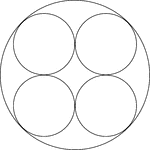# 4 circles inside a smaller circle. Radius calculation

#### MathsFormula

##### Junior Member
A large circle has a radius of 10 cm. Contained within this circle are four smaller circles of equal size (fitting inside the larger circle exactly). The question asks: Find the radius of the largest circle which will fit in the middle.

Here is a link to a picture that I’ve pulled from Google that shows the same diagram as the maths book:The book answer is: 1.72 cm

My attempt: The distance between the points of touch of the smaller circles is: 20/3 = 6.66 cm
So the distance between the point of touch of one of the smaller circles and the centre of the larger circle is: 6.66/2 = 3.33 cm
Therefore the radius of the smaller circle should also be 3.33 cm
Total diameter of two circles facing diagonally opposite each other is 3.33 x 4 = 13.32 cm

So the distance remaining between the circles is 20 – 13.32 = 6.68 cm

So radius if a circle in the middle would be 6.68/2 = 3.34 cm THIS IS THE WRONG ANSWER

Last edited by a moderator:

#### jonah2.0

##### Junior Member
DISCLAIMER: Beer soaked rambling/opinion/observation/reckoning ahead. Read at your own risk. Not to be taken seriously. In no event shall the wandering math knight-errant Sir jonah in his inebriated state be liable to anyone for special, collateral, incidental, or consequential damages in connection with or arising out of the use of his beer (and tequila) powered views.

Sorry having problems posting and made many mistakes in the post above + can't edit. So re-posting now:

A large circle has a radius of 10 cm. Contained within this circleare four smaller circles of equal size (fitting inside the larger circle exactly).The question asks : find the radius of the largest circle which will fit in themiddle.

Here is a link to a picture that I’ve pulled from Google thatshows the same diagram as the maths book: http://etc.usf.edu/clipart/42900/42919/circle-25_42919_mth.gif
The book answer is : 1.72 cm

My attempt: The distance between the points of touch of thesmaller circles is : 20/3 = 6.66 cm
So the distance between the point of touch of one of the smallercircles and the centre of the larger circle is : 6.66/2 = 3.33 cm
Therefore the radius of the smaller circle should also be3.33 cm
Total diameter of two circles facing diagonally oppositeeach other is 3.33 x 4 = 13.32 cm

So the distance remaining between the circles is 20 – 13.32= 6.68 cm

So radius if a circle in the middle would be 6.68/2 = 3.34cm THIS IS THE WRONG ANSWER
Had a hard time visualizing the solution for this one.
With a little help from our good friend Google at
http://www.algebra.com/algebra/homework/Circles/Circles.faq.question.308572.html
it would seem that the book answer of 1.72 cm. is correct.
You should get 10-2(10*2^.5-10) or 30-20*2^.5

Last edited:

#### jonah2.0

##### Junior Member
DISCLAIMER: Beer soaked rambling/opinion/observation/reckoning ahead. Read at your own risk. Not to be taken seriously. In no event shall the wandering math knight-errant Sir jonah in his inebriated state be liable to anyone for special, collateral, incidental, or consequential damages in connection with or arising out of the use of his beer (and tequila) powered views.
Googling "circle inscribed in a quarter circle" led me to a similar problem here nearly 8 years ago at

#### MathsFormula

##### Junior Member
Thanks

Thanks Dennis. This really helped:

u = radius of the 4 circles

I still need a little more help with the algebra please. I don’t know where I’vegone wrong with my calculation.

As Denis said:

u = radius of the 4 circles

Radius of large circle = 2u + v = 10

v = 10 - 2u ....... (i)

A square forms with sides of length u.
It can be split into a triangle with hypotenuse of length u+v

So u2 + u2 = (u + v)2

METHOD A

2u2 = (u + v) (u +v)
2u2 = u2+ uv +uv + v2
u2 =2uv + v2 …… (ii)

Inserting (i) into (ii) :
u2 = 2u (10-2u) +(10-2u)2
u2 = 20u - 4u2 +(10-2u)2
5u2 – 20u = (10-2u) (10-2u)
5u2 – 20u = 100 -20u – 20u + 4u2
u2 =100 – 20u….. NOW WHAT DO I DO?I’m stuck

I’ve also tried a different method:
METHOD B
Earlier I said u2+ u2 = (u + v)2
So 2u2= (u + v)2
Square rooting both sides of the equation gives:
u2 = u + v
1.414u = u + v
0.414 = v

v = 10 - 2u ....... (i)
u = (10-v)/2
u = (10-0.414)/2